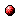# On algorithms for a binary-real (max,×) matrix approximation problem

Reference:
B. De Schutter, J. Schepers, and I. Van Mechelen, "On algorithms for a binary-real (max,×) matrix approximation problem," Proceedings of the 45th IEEE Conference on Decision and Control, San Diego, California, pp. 5168-5173, Dec. 2006.

Abstract:
We consider algorithms to solve the problem of approximating a given matrix D with the (max,×) product of a binary (i.e., a 0-1) matrix S and a real matrix P: minS,P || S · P - D ||. The norm to be used is the l1, l2 or l norm, and the (max,×) matrix product is constructed in the same way as the conventional matrix product, but with addition replaced by maximization. This approximation problem arises among others in data clustering applications where the maximal component instead of the sum of the components determines the final result. We propose several algorithms to address this problem. The binary-real (max,×) matrix approximation problem can be solved exactly using mixed-integer programming, but since this approach suffers from combinatorial explosion we also propose some alternative approaches based on alternating nonlinear optimization, and a method to obtain good initial solutions. We conclude with a simulation study in which the performance and optimality of the different algorithms are compared.Corresponding technical report: pdf file (136 KB)
Note: More information on the pdf file format mentioned above can be found here.

Bibtex entry:

@inproceedings{DeSSch:06-027,
author={B. {D}e Schutter and J. Schepers and I. {V}an Mechelen},
title={On algorithms for a binary-real $(\max,\times)$ matrix approximation problem},
booktitle={Proceedings of the 45th IEEE Conference on Decision and Control},